# The ROBUSTREG Procedure

### MM Estimation

Subsections:

MM estimation is a combination of high breakdown value estimation and efficient estimation that was introduced by Yohai (1987). It has the following three steps:

1. Compute an initial (consistent) high breakdown value estimate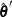. The ROBUSTREG procedure provides two kinds of estimates as the initial estimate: the LTS estimate and the S estimate. By default, the LTS estimate is used because of its speed and high breakdown value. The breakdown value of the final MM estimate is decided by the breakdown value of the initial LTS estimate and the constant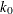in the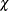function. To use the S estimate as the initial estimate, specify the INITEST=S option in the PROC ROBUSTREG statement. In this case, the breakdown value of the final MM estimate is decided only by the constant. Instead of computing the LTS estimate or the S estimate as the initial estimate, you can also specify the initial estimate explicitly by using the INEST= option in the PROC ROBUSTREG statement. For more information, see the section INEST= Data Set.

2. Find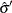such that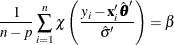where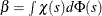.

The ROBUSTREG procedure provides two choices for: Tukey’s bisquare function and Yohai’s optimal function.

Tukey’s bisquare function, which you can specify by using the option CHIF=TUKEY, is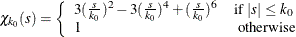wherecan be specified by using the K0= option. The defaultis 2.9366, such that the asymptotically consistent scale estimatehas a breakdown value of 25%.

Yohai’s optimal function, which you can specify by using the option CHIF=YOHAI, is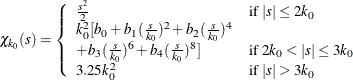where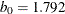,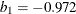,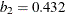,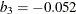, and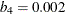. You can use the K0= option to specify. The defaultis 0.7405, such that the asymptotically consistent scale estimatehas a breakdown value of 25%.

3. Find a local minimum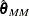of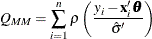such that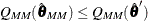. The algorithm for M estimation is used here.

The ROBUSTREG procedure provides two choices for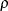: Tukey’s bisquare function and Yohai’s optimal function.

Tukey’s bisquare function, which you can specify by using the option CHIF=TUKEY, is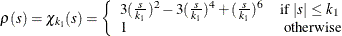where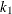can be specified by using the K1= option. The defaultis 3.440 such that the MM estimate has 85% asymptotic efficiency with the Gaussian distribution.

Yohai’s optimal function, which you can specify by using the option CHIF=YOHAI, is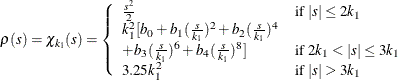wherecan be specified by using the K1= option. The defaultis 0.868 such that the MM estimate has 85% asymptotic efficiency with the Gaussian distribution.

#### Algorithm

The initial LTS estimate is computed using the algorithm described in the section LTS Estimate. You can control the quantile of the LTS estimate by specifying the option INITH=h, where h is an integer between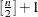and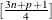. By default,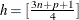, which corresponds to a breakdown value of around 25%.

The initial S estimate is computed using the algorithm described in the section S Estimate. You can control the breakdown value and efficiency of this initial S estimate by the constant, which you can specify by using the K0= option.

The scale parameter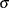is solved by an iterative algorithm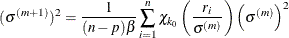where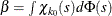.

After the scale parameter is computed, the iteratively reweighted least squares (IRLS) algorithm with fixed scale parameter is used to compute the final MM estimate.

#### Convergence Criteria

In the iterative algorithm for the scale parameter, the relative change of the scale parameter controls the convergence.

In the iteratively reweighted least squares algorithm, the same convergence criteria for the M estimate that are used before are used here.

#### Bias Test

Although the final MM estimate inherits the high breakdown value property, its bias from the distortion of the outliers can be high. Yohai, Stahel, and Zamar (1991) introduced a bias test. The ROBUSTREG procedure implements this test when you specify the BIASTEST= option in the PROC ROBUSTREG statement. This test is based on the initial scale estimateand the final scale estimate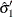, which is the solution of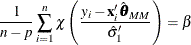Let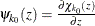and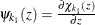. Compute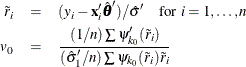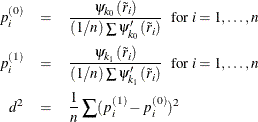Let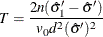Standard asymptotic theory shows that T approximately follows a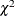distribution with p degrees of freedom. If T exceeds thequantile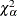of thedistribution with p degrees of freedom, then the ROBUSTREG procedure gives a warning and recommends that you use other methods. Otherwise, the final MM estimate and the initial scale estimate are reported. You can specifyby using the ALPHA= option after the BIASTEST= option. By default, ALPHA=0.99.

#### Asymptotic Covariance and Confidence Intervals

Because the MM estimate is computed as an M estimate with a known scale in the last step, the asymptotic covariance for the M estimate can be used here for the asymptotic covariance of the MM estimate. Besides the three estimators H1, H2, and H3 as described in the section Asymptotic Covariance and Confidence Intervals, a weighted covariance estimator H4 is available. H4 is calculated as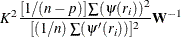where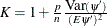is the correction factor and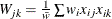,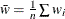.

You can specify these estimators by using the option ASYMPCOV=[H1 | H2 | H3 | H4]. The ROBUSTREG procedure uses H4 as the default. Confidence intervals for estimated parameters are computed from the diagonal elements of the estimated asymptotic covariance matrix.

#### R Square and Deviance

The robust version of R square for the MM estimate is defined as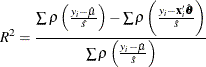and the robust deviance is defined as the optimal value of the objective function on the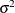scale,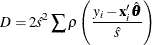where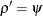,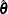is the MM estimator of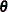,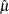is the MM estimator of location, andis the MM estimator of the scale parameter in the full model.

#### Linear Tests

For MM estimation, you can use the sametest and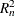test that used for M estimation. For more information, see the section Linear Tests.

#### Model Selection

For MM estimation, you can use the same two model selection methods that are used for M estimation. For more information, see the section Model Selection.